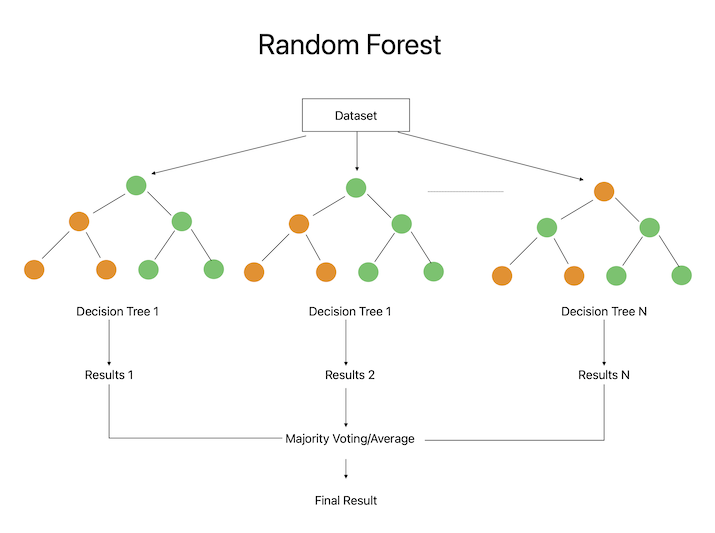# Does label encoding affect tree-based algorithms?

Regression and classification are two common uses for tree-based algorithms, which are popular machine-learning techniques. Gradient boosting, decision trees, and random forests are a few examples of common tree-based techniques.These algorithms can handle data in both categories and numbers. Nonetheless, prior to feeding the algorithm, categorical data must be translated into a numerical form. One such strategy is label encoding. In this blog post, we'll examine how label encoding impacts tree-based algorithms.

## What is Label Encoding?

Label encoding is a typical machine-learning approach for transforming categorical input into numerical data. It entails giving each category in the dataset a different integer value. When using machine learning algorithms that need numerical data as input, this strategy is helpful. Label encoding can affect how well the model performs, thus it's vital to use it carefully. Label encoding produces less-than-ideal results because it implies a natural ordering of categories that might not hold true in practice. Thus, when working with categorical data, one-hot encoding is advised over label encoding.

Tree-based algorithms can handle categorical data by separating it into categories. The algorithm chooses the characteristic that produces the most information by dividing the input into its component parts. The information gain quantifies how much information a feature adds to the target variable. But, categorical data must first be converted to numerical data before being provided to the algorithm.

## Effect of Label Encoding on Tree-Based Algorithms

Label encoding is a straightforward method that is simple to use. Nonetheless, it could affect how well-tree-based algorithms function. Let's investigate how gradient boosting, random forests, and decision trees respond to label encoding.

## Decision Tree

Decision trees are built by recursively separating data depending on the most informative attribute. The decision tree algorithm assesses the quality of each split using criteria. Gini impurity is one such standard. The Gini impurity calculates the likelihood of misclassifying a sample taken at random. The algorithm's objective is to reduce the Gini impurity at every split.

The effectiveness of decision trees can be impacted by label encoding. Imagine a categorical feature "Size" with values "Small", "Medium", and "Big". If we give the categories the numbers 0, 1, and 2, the algorithm could believe that the categories are naturally arranged in that order. Being the average of "Little" and "Big," the algorithm can regard "Medium" as being. The decision tree might not function well since this assumption might not hold true in practice.

## Random Forest

The ensemble learning method known as random forests uses many decision trees to improve the performance of the model. Each decision tree is trained using a different set of characteristics and data. The ultimate prognosis is calculated by averaging the forecasts each tree provides.

Similar to decision trees, label encoding can influence the performance of random forests. The method can perform poorly if it assumes that there is an ordering even while the categories don't naturally have one. The use of one-hot encoding as opposed to label encoding is one technique to address this problem. Each category is represented as a binary vector with a length equal to the number of categories in one-hot encoding. For example, the feature "Color" with the categories "Red," "Green," and "Blue" would be represented by [1, 0, 0], [0, 1, 0], and [0, 0, 1].

A strong learner is produced by combining weak learners using the iterative gradient boosting process. Weak learners are taught on the residuals of stronger learners throughout each repetition. The aggregate of each learner's individual guesses makes up the final forecast.

Similar to random forests, label encoding can have an impact on how well gradient boosting performs. Without a natural ordering for the categories, the algorithm can make incorrect decisions and perform poorly. By switching to one-hot encoding from label encoding, this can possibly be avoided.

It's crucial to keep in mind that label encoding can be effective for categorical characteristics with a natural ordering. For instance, if the category "Education Level" has the values "High School," "Bachelor's Degree," "Master's Degree," and "PhD," we can give each value 0, 1, 2, and 3, respectively. As the categories are naturally arranged in this situation, label encoding makes sense.

## Conclusion

In conclusion, the performance of tree-based algorithms can be impacted by label encoding, particularly when the categories lack a natural ordering. In these circumstances, one-hot encoding is a preferable substitute. Nonetheless, categorical characteristics with a natural ordering can benefit from label encoding. As usual, it's crucial to test out many strategies to see which one solves your specific issue the best.

Updated on: 25-Apr-2023

508 Views